The Theory \ Details \ portfolio optimization \ risk aversion

# Portfolio Optimization

## Measures of risk aversion

For the classification of utility functions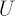it is efficient to use special measures reflecting character and degree of investor’s risk aversion. Most common are two types of such measures: Absolute Risk Aversion Coefficient and Relative Risk Aversion Coefficient.

### Absolute Risk Aversion and CARA Utility Functions

Illustrative Example
Assume Total investor’s Wealth is equal to \$1000. Out of this the investor is willing to risk \$500. How much money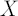investor will be willing to risk when his/her Total Wealth reaches \$2000?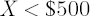corresponds to Increasing Absolute Risk Aversion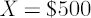corresponds to Constant Absolute Risk Aversioncorresponds to Decreasing Absolute Risk Aversion

Note. Natural assumption is that most investors have decreasing absolute risk aversion.

Definition. Absolute Risk Aversion Coefficient at pointis defined as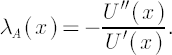Utility functions with Constant Absolute Risk Aversion Coefficient are called CARA Utility Functions.

Example of CARA utility function
Exponential utility functions: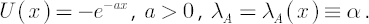### Relative Risk Aversion and CRRA utility functions

Illustrative Example
Assume that we are once again in the framework of the previous example.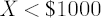corresponds to Increasing Relative Risk Aversion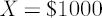corresponds to Increasing Relative Risk Aversion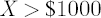corresponds to Increasing Relative Risk Aversion

Note. Most often investors are assumed to have Constant Relative Risk Aversion

Definition. Relative Risk Aversion Coefficient at pointis defined as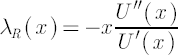. Utility functions with Constant Relative Risk Aversion Coefficient are called CRRA Utility Functions

Examples of CRRA Utility Functions

1. Power utility functions: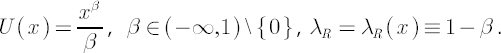2. Logarithmic utility functions:Note. As a rule, existing methods of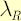estimation, when applied to real-world situations, produce results
in range from 2 to 4.

Key Result

Maximization of expected value of CRRA utility from portfolio’s terminal wealth leads to portfolio strategies
with constant portfolio weights over time.

Note. SmartFolio optimization module handles portfolio strategies with constant portfolio weights
only. The above result justifies this approach.